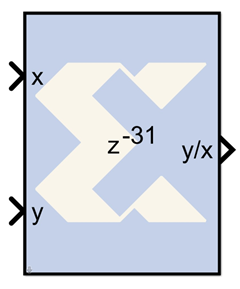# CORDIC DIVIDER - 2020.2 English

## Vivado Design Suite Reference Guide: Model-Based DSP Design Using System Generator (UG958)

Document ID
UG958
Release Date
2020-11-18
Version
2020.2 English

The Xilinx CORDIC DIVIDER reference block implements a divider circuit using a fully parallel CORDIC (COordinate Rotation DIgital Computer) algorithm in Linear Vectoring mode.That is, given a input <x,y>, it computes the output y/x. The CORDIC processor is implemented using building blocks from the Xilinx blockset.

The CORDIC divider algorithm is implemented in the following 4 steps:

1. Co-ordinate Rotation - The CORDIC algorithm converges only for positive values of x. The input vector is always mapped to the 1st quadrant by making the x and y coordinate non-negative. The divider circuit has been designed to converge for all values of X and Y, except for the most negative value.
2. Normalization - The CORDIC algorithm converges only for y less than or equal to 2x. The inputs x and y are shifted to the left until they have a 1 in the most significant bit (MSB). The relative shift of y over x is recorded and passed on to the co-ordinate correction stage.
3. Linear Rotations - For ratio calculation, the resulting vector is rotated through progressively smaller angles, such that y goes to zero. In the final stage, the rotation yields y/x.
4. Co-ordinate Correction - Based on the co-ordinate axis and a relative shift applied to y over x, this step assigns the appropriate sign to the resulting ratio and multiplies it with 2^(relative shift of y over x).

## Block Parameters

The block parameters dialog box can be invoked by double-clicking the icon in your Simulink model.

Parameters specific to this reference block are as follows:

• Number of Processing Elements specifies the number of iterative stages used for linear rotation.
• X,Y Data Width: specifies the width of the inputs x and y. The inputs x and y should be signed data type with the same data width.
• X,Y Binary Point Position: specifies the binary point position for inputs x and y. The inputs x and y should be signed data type with the same binary point position.
• Latency for each Processing element: This parameter sets the pipeline latency after each iterative linear rotation stage.

The latency of the CORDIC divider block is calculated based on the formula specified as follows: Latency = 4 + data width + sum (latency of Processing Elements)

## Reference

1. J. E. Volder, The CORDIC Trigonometric Computing Technique, IRE Trans. On Electronic Computers, Vol. EC-8, 1959, pp. 330-334.
2. J. S. Walther, A Unified Algorithm for Elementary Functions, Spring Joint Computer Conference (1971) pp. 379-385.
3. Yu Hen Hu, CORDIC-Based VLSI Architectures for Digital Signal Processing, IEEE Signal Processing Magazine, pp. 17-34, July 1992.